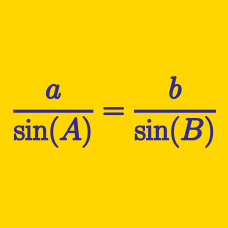Geometry

# Cosine Rule

If triangle $$ABC$$ has side lengths $$a=7$$, $$b=12$$ and $$c=11$$, the value of $$\cos A$$ can be expressed as $$\frac{p}{q}$$, where $$p$$ and $$q$$ are coprime positive integers. What is the value of $$p+q$$?

Details and assumptions

$$a$$, $$b$$ and $$c$$ are the lengths of the sides opposite to the vertices $$A$$, $$B$$ and $$C$$, respectively.

In a triangle, an angle of $$60^\circ$$ is formed by two sides of lengths $$4$$ and $$11$$. If the length of the remaining side is $$\sqrt{a}$$, what is $$a$$?

If parallelogram $$ABCD$$ has $$\overline{AB}=8$$, $$\overline{BC}=4$$ and $$\angle ABC=60^{\circ}$$, what is the square of the length of the diagonal $$\overline{BD}$$?

Let $$ABC$$ be a triangle such that $$\angle A = 30^{\circ}$$, $$a = 9 \sqrt{3}$$ and $$c = 18 \sqrt{3}$$. What is the value of $$b$$?

Details and assumptions

$$a$$, $$b$$ and $$c$$ are the lengths of the sides opposite to the vertices $$A$$, $$B$$ and $$C$$, respectively.

If triangle $$ABC$$ has $$\angle A = 45^{\circ}$$, $$b=28$$ and $$c=\sqrt{6}+14\sqrt{2}$$, what is the value of $$a^2$$?

Details and assumptions

$$a$$, $$b$$ and $$c$$ are the lengths of the sides opposite to the vertices $$A$$, $$B$$ and $$C$$, respectively.

×

Problem Loading...

Note Loading...

Set Loading...